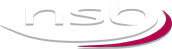# Northampton School for Boys

•••••••# Maths

### Team Leader - Mr M Mirnateghi

Students in Years 7, 8, 9, 10, and 11 are taught in one of three ability bands and study a course delivered in modules. Each module of work concludes with a test to ensure that students are competent in all aspects of those topics. The modules are delivered by the Team using a wide variety of teaching and learning styles. Students’ work includes learning activities, consolidation and practice work, problem-solving and investigative work.

There is an emphasis on activities which increase both confidence and skills. A wide range of software is used to support the delivery of the Scheme of Work. The Mathematics Team has access to rooms that are equipped with computers as well as electronic whiteboards and their use is an integral part of all our students' work.  UKMT individual and team challenges are used at all ages.

#### KS3

Students in KS3 start to use ICT skills to enhance and reinforce mathematical concepts. They are encouraged to develop independent learning, problem solving, and elementary research skills in a wider context. In addition to the revision resources on http://extranet.nsb.northants.sch.uk.  The Mathematics department currently subscribes to Mymaths and students are routinely set homework tasks from this website. Students are also encouraged to use the following free online resources;
GeoGebra, Constructions, Examsolutions, Mental Arithmetic.

Term 1
Number 1: BODMAS. Multiplication and division by powers of 10.
Algebra 1: Collecting like terms. Simple substitution. Multiplying out brackets.
Shape & Space 1: Angle properties of triangles and quadrilaterals. Angles and Parallel lines.

Term 2
Number 2: Calculations involving fractions, decimals and percentages.
Algebra 2: Forming and solving simple equations.
Shape & Space 2: Construction of triangles and Loci. Constructions

Term 3
Handling Data 1: Collecting and displaying data.
Graphs 1:  Simple straight lines. Travel graphs. Sets 1/2: Work on y = mx + c. GeoGebra.

Term 4
Handling Data 2: Mean, mode, median, range. Probability. Venn diagram.
Number 3: Types of number, linear sequences, indices.

Term 5
Shape & Space 3: Angle properties of polygons. Circumference and area of circles.
Number 4: Percentage increase and decrease.

Term 6
Number 5: Ratio and proportion.
Shape & Space 4: Area and volume.
Algebra 3: Further work with equations.

#### Year 8

Term 1
Number 1: Rules of indices.
Algebra 1: Expecting brackets and factorising.

Term 2
Number 2: Fractions, decimals and percentages.
Shape & Space 2: Angles in parallel lines. Transformations.

Term 3
Handling Data 3: Probability for single and combined events.
Graphs 1: Linear and quadratic graphs. GeoGebra

Term 4
Handling Data 2: Mean, mode and median for frequency and grouped data.
Number 3: Ratio and proportion.

Term 5
Shape & Space 3: Pythagras’ Rule, trigonometry.
Revision for the End of Year test.

Term 6
Number 4: Further work with fractions, decimals and percentages.
Algebra 2: Further work on solving equations and factorising.

#### Year 9

Term 1
Number 1: All rules of indices. Rounding off to significant figures.
Algebra 1: Solving equations involving fractions.

Term 2
Number 2: Fractions, decimals and percentages.
Shape & Space 1: Angles in polygons. Trigonometry. Similar shapes.

Term 3
Handling Data 3: Probability for single and combined events.
Graphs 1: Linear and quadratic graphs.  Finding the equation of a line. GeoGebra
Algebra 2: Solving simultaneous equations.

Term 4
Handling Data 2: Cumulative frequency graph. Box plot.  Stem and leaf table.
Number 3: Ratio and proportion.
Algebra 3: Factorising and solving quadratics.

Term 5
Revision for SATs and feedback.
Extension work and problem solving.

Term 6
Shape & Space 2: Circle theorems. Advanced trigonometry. Exact values of trig ratios.
Algebra 2: Further work on solving quadratics using the formula.

#### Year 10 & 11

At KS4 the Team currently offers the Edexcel GCSE Mathematics course. Sets 1and 2 sit for the Higher Tier papers and Set 3 sit for the Foundation Tier papers. Full details of the specification and units of content can be found on the Edexcel website here

#### Year 10

Term 1: Number Work

Term 2: Algebra

Term 3: Graphs

Term 4: Handling Data

Term 5: Shape and Space

Term 6: Proof/Transformations/Vectors

Year 11 will comprise mainly of revision of work covered in Year 10 and extension of these topics. Problem solving questions will be worked on. All groups will also sit 3 Mock exams in late November, January and March.

Useful resources for revision are:

www.mathsgenie.co.uk

Also, practice papers can be found on the school VLE.

#### Year 12 & 13

At KS5 students will follow a linear Edexcel course with 3 examinations at the end of the 2 years. Two papers are examined on Pure Mathematics and one paper is on statistics and mechanics combined.

The aims and objectives of studying A Level Mathematics are to enable students to understand mathematics and mathematical processes in a way that promotes confidence, fosters enjoyment and provides a strong foundation for progress to further study. It extends their range of mathematical skills and techniques and helps them to understand coherence and progression in mathematics and how different areas of mathematics are connected. The students use their mathematical skills and techniques to solve challenging problems that require them to decide on the solution strategy and they take increasing responsibility for their own learning and the evaluation of their own mathematical development.

Full details of the specification and units of content can be found on Edexcel website:

#### Mathematics Team Mr M. Mirnateghi Mr M. Quinn Mrs S. Bradley-Brophy Mr J. Sinyoro Mr M. Edwards Mrs S. Minai Mr P. Livingstone Mr D. Pearle Mr L. Barker Mrs N. Kwei-Tagoe Mrs K. Wilkins Mr J. Easton

Northampton School for Boys, Billing Road, Northampton, NN1 5RT
01604 230240